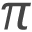# User Guide 3.3

• Other Versions

# Math Expressions

Math Expressions (i.e. mathematical operators), or formulae, can be employed in Interactions by using the Formula Editor. The Formula Editor is a WYSIWYG editor based on MathQuill, which allows you to use mathematical symbols to create LaTeX expressions containing mathematical operators. It is found in the Custom Interactions section.

To access the Formula Editor follow the steps below.

1. Once you have created a new Item, click on the Custom Interactions Library on the left, and drag the Math Entry icon onto the blank item and drop it onto the Canvas.

The MathQuill editor can also be accessed from any block by selecting Insert Math Expressionand then clicking on the WYSIWYG editor.

A list of mathematical symbols will appear, with an empty text field below.